# Suppose that the matrix A A has the following eigenvalues and eigenvectors: (1 point) Suppose that...

Suppose that the matrix A A has the following eigenvalues and eigenvectors: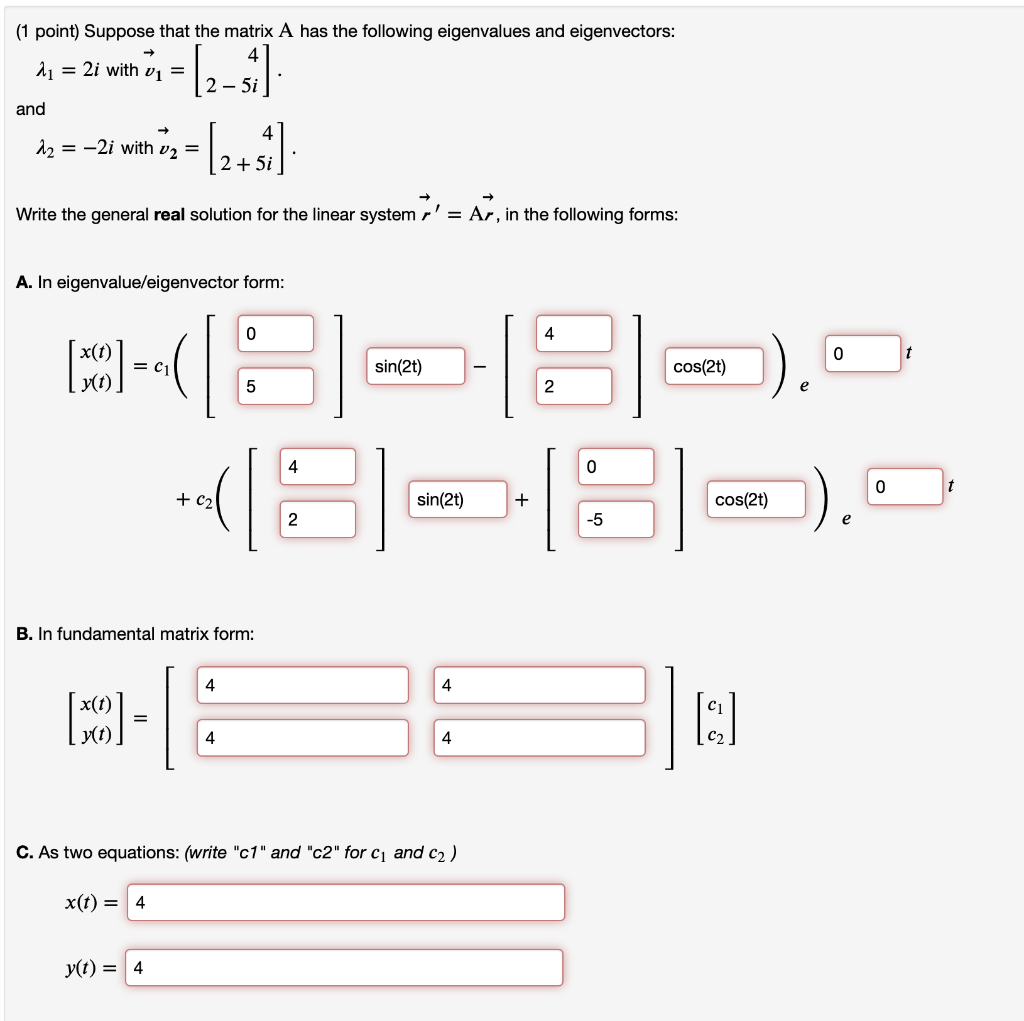(1 point) Suppose that the matrix A has the following eigenvalues and eigenvectors: 2 = 2i with v1 = 2 - 5i and - 12 = -2i with v2 = (2+1) 2 + 5i Write the general real solution for the linear system r' = Ar, in the following forms: A. In eigenvalue/eigenvector form: 0 4 0 t MODE = C1 sin(2t) cos(2) 5 2 4 0 0 t + C2 sin(2t) + cos(2) 2 -5 B. In fundamental matrix form: 4 4 x(t) [CO] Is 4 4 C. As two equations: (write "c1" and "c2" for ci and c2) x(t) = 4 yt) = 4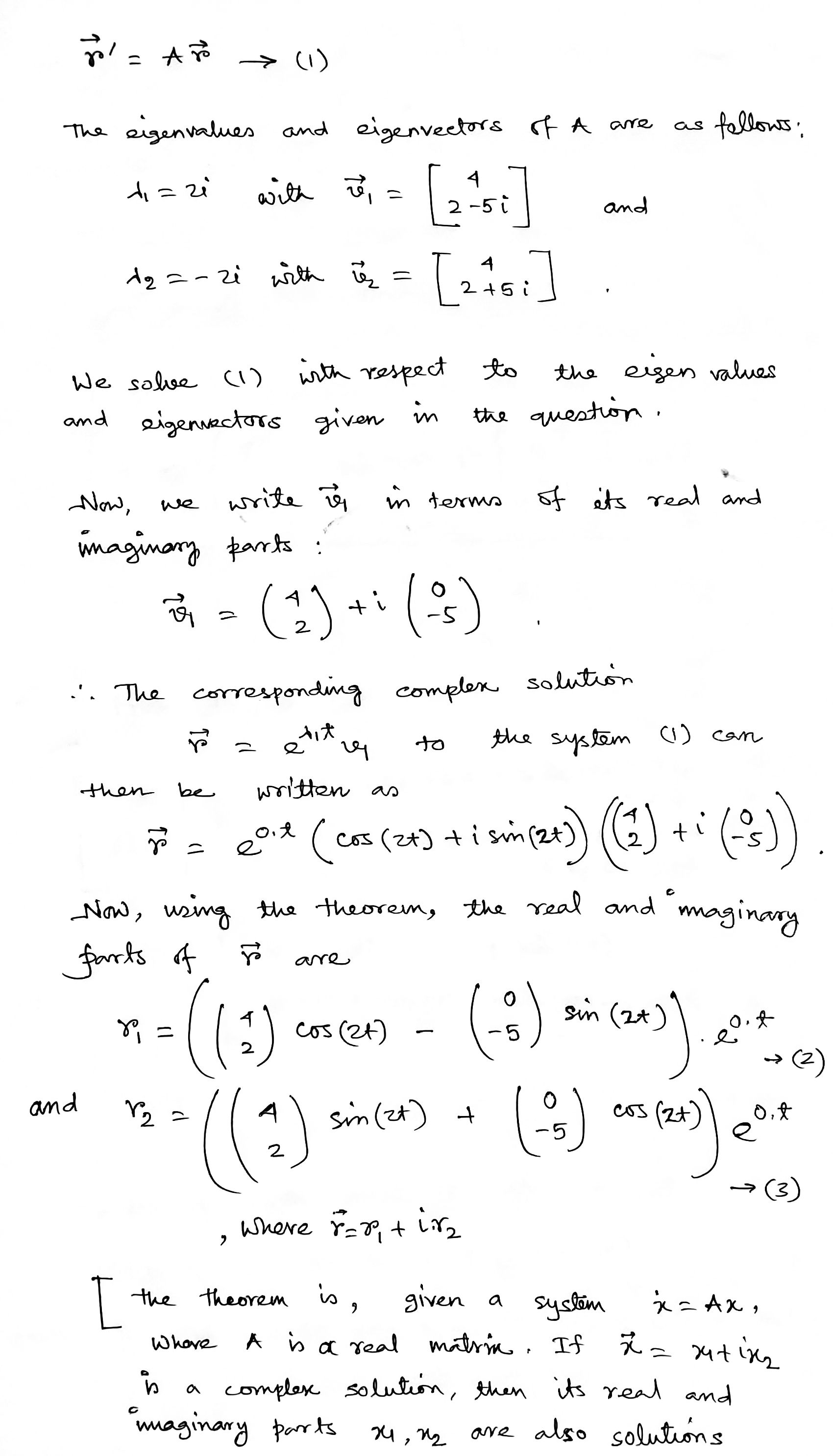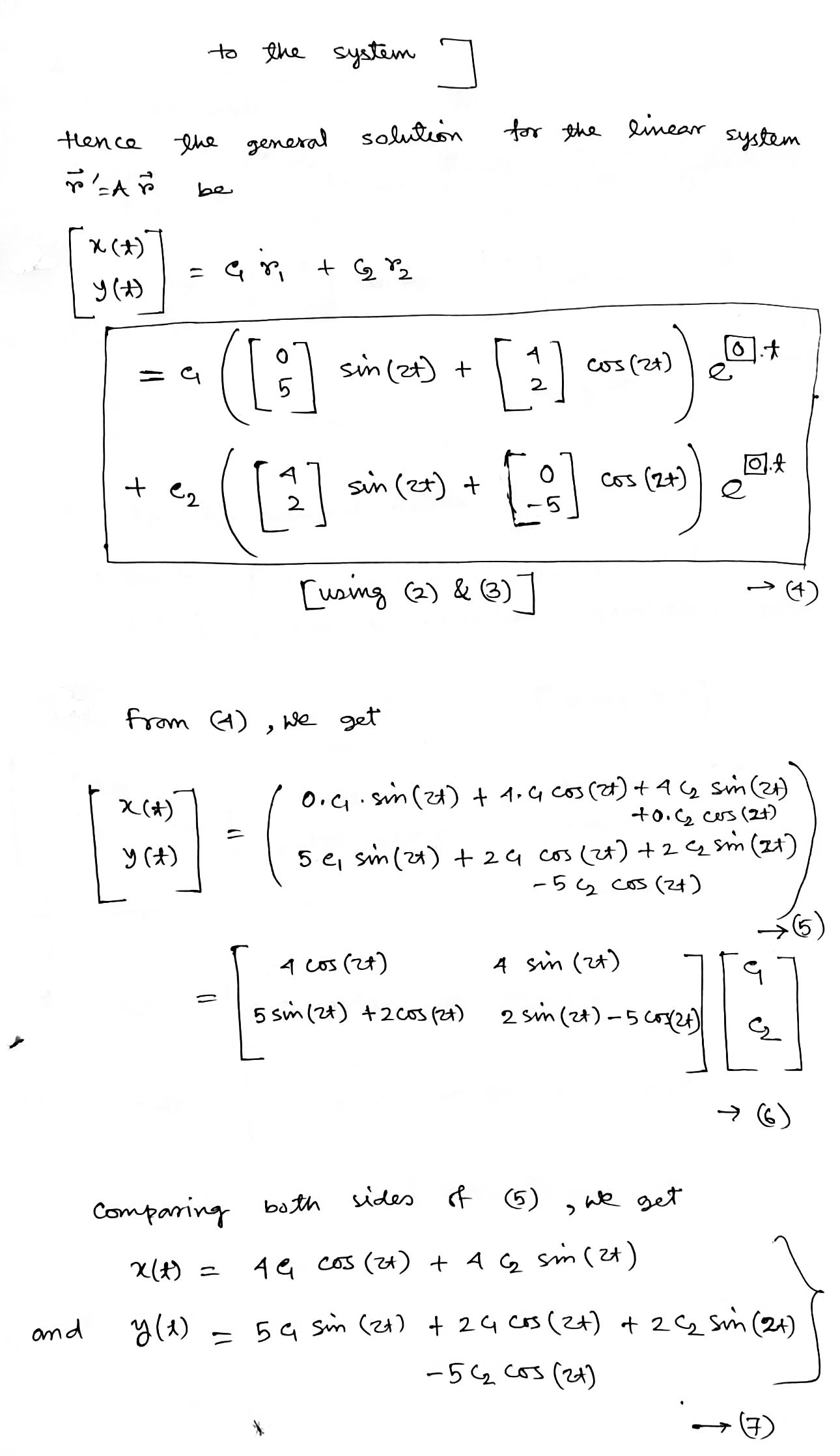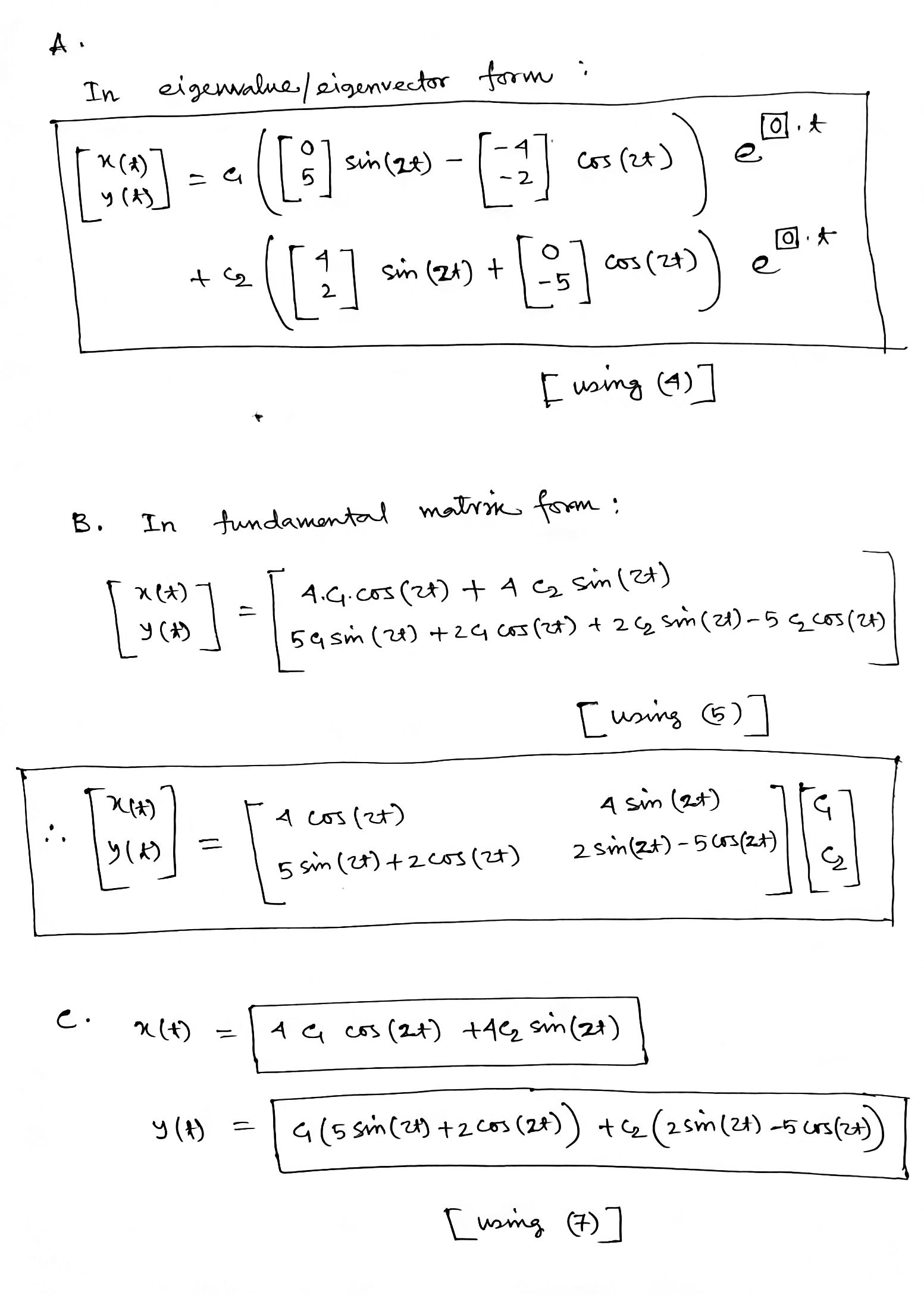Please give a thumbs up if you are benefited.

##### Add Answer of: Suppose that the matrix A A has the following eigenvalues and eigenvectors: (1 point) Suppose that...
Similar Homework Help Questions
• ### (1 point) Suppose that the matrix A has the following eigenvalues and eigenvectors: 4 = 2...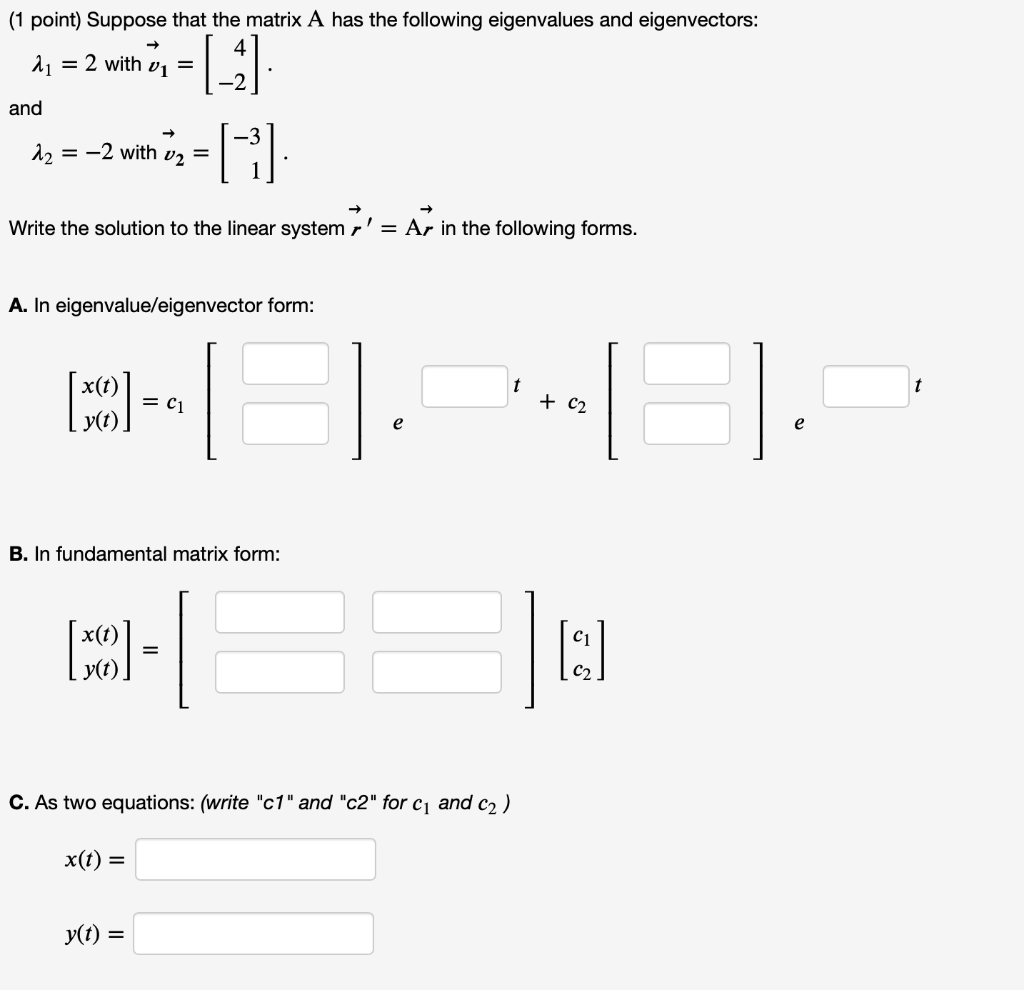(1 point) Suppose that the matrix A has the following eigenvalues and eigenvectors: 4 = 2 with vi = and |_ G 12 = -2 with v2 = Write the solution to the linear system r' = Ar in the following forms. A. In eigenvalue/eigenvector form: x(t) (50) = C1 + C2 e e B. In fundamental matrix form: (MCO) = I: C. As two equations: (write "c1" and "c2" for C1 and c2) x(t) = yt) =

• ### (1 point) Suppose that the matrix A has the following eigenvalues and eigenvectors: A1 = 4...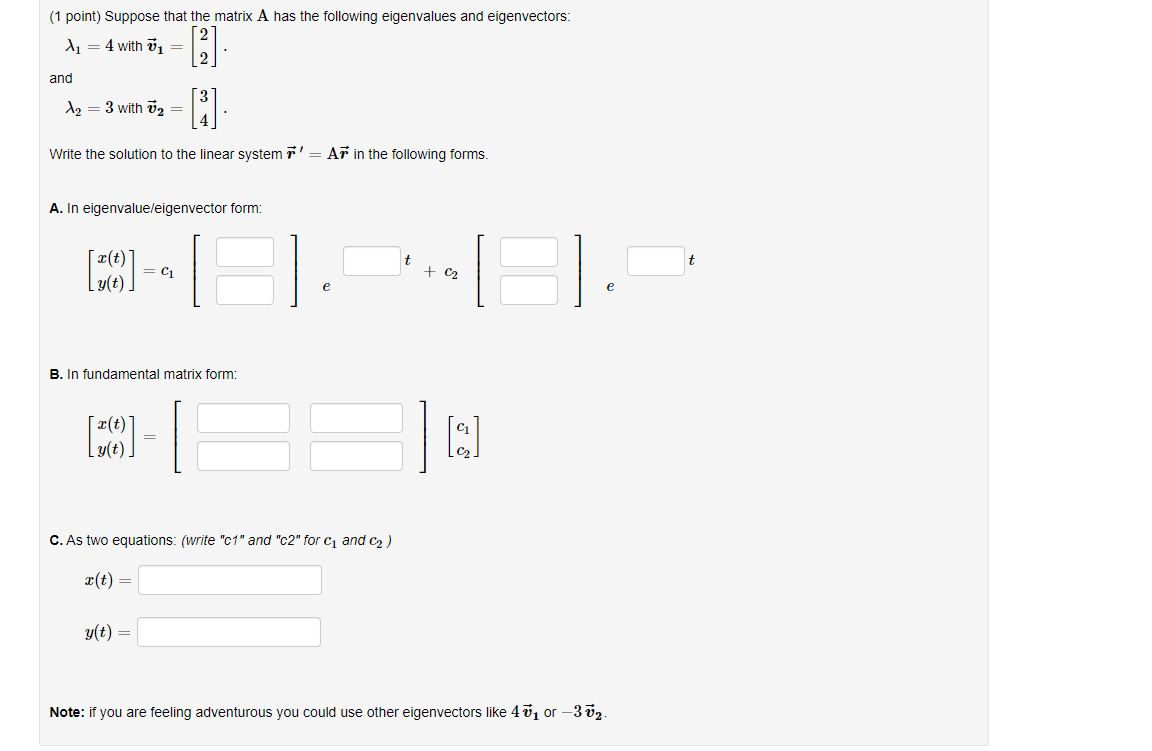(1 point) Suppose that the matrix A has the following eigenvalues and eigenvectors: A1 = 4 with = and  [i] Az = 3 with Ū2 = Write the solution to the linear system r' = Ar in the following forms. A. In eigenvalue/eigenvector form: t (10) -- + C2 e e B. In fundamental matrix form: (39) - g(t). C. As two equations: (write "c1" and "c2" for C and C2) X(t) = g(t) = Note: if you are...

• ### (1 point) Suppose that the matrix A has the following eigenvalues and eigenvectors 2-2i and -2+2i Write the solution to...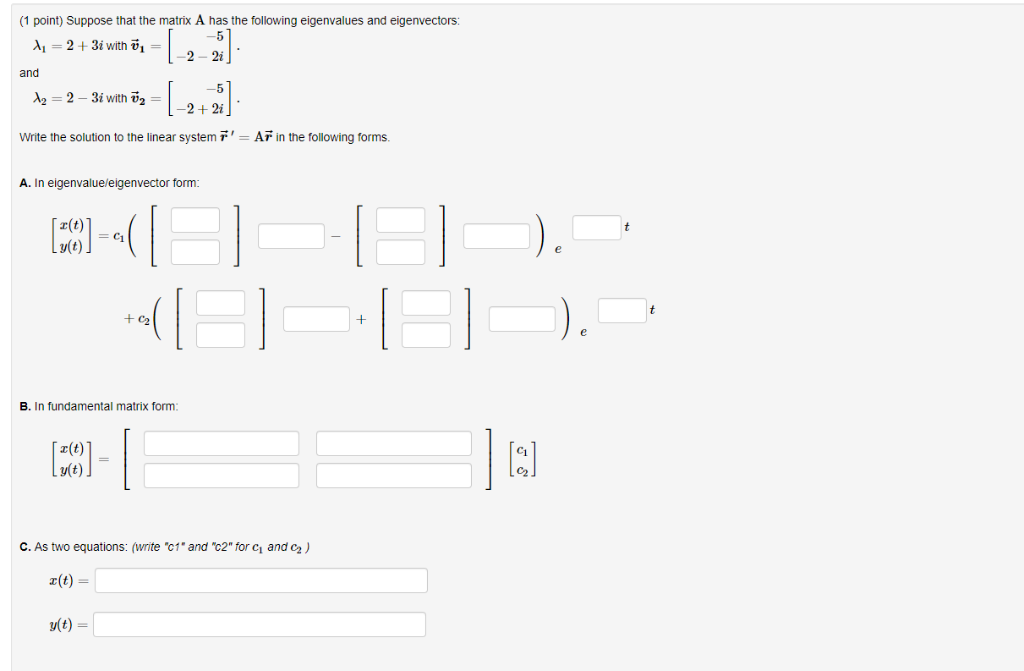(1 point) Suppose that the matrix A has the following eigenvalues and eigenvectors 2-2i and -2+2i Write the solution to the linear system AF in the following forms A. In eigenvalueleigenvector form r(t) B. In fundamental matrix form z(t) v(t) C. As two equations: (write "c1* and "c2" for ci and C2) a(t)- v(t)- (1 point) Suppose that the matrix A has the following eigenvalues and eigenvectors 2-2i and -2+2i Write the solution to the linear system AF in the...

• ### (1 point) Suppose that the matrix A has repeated eigenvalue with the following eigenvector and generalized...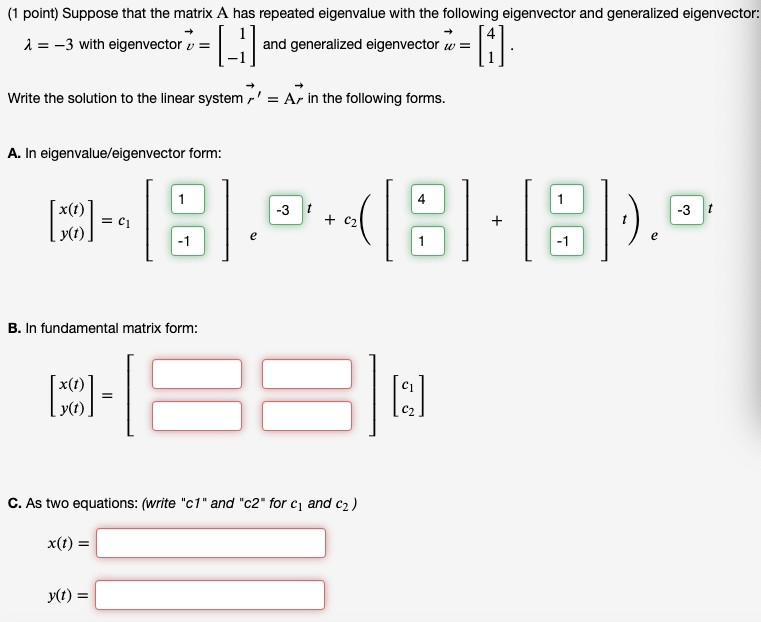(1 point) Suppose that the matrix A has repeated eigenvalue with the following eigenvector and generalized eigenvector: i = -3 with eigenvector v = and generalized eigenvector w= = [-] =  Write the solution to the linear system r' = Ar in the following forms. A. In eigenvalue/eigenvector form: 1 (O) = - 18.05.8:8)... y(t) B. In fundamental matrix form: (O)- x(1) y(t) [:] C. As two equations: (write "c1" and "c2" for c and c2) X(t) = yt)...

• ### (1 point) Suppose that the matrix A has repeated eigenvalue with the following eigenvector and generalized...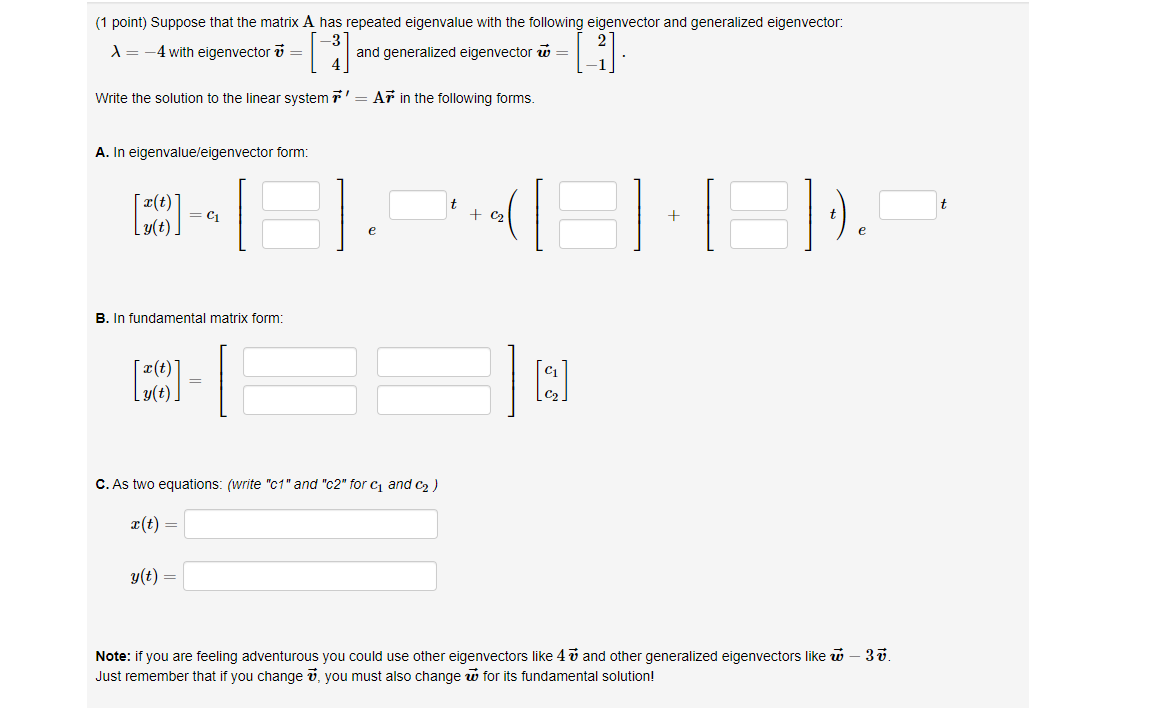(1 point) Suppose that the matrix A has repeated eigenvalue with the following eigenvector and generalized eigenvector: X= -4 with eigenvector v = and generalized eigenvector ū= [] (-1) Write the solution to the linear system r' = Ar in the following forms. A. In eigenvalue/eigenvector form: t t [CO] = C1 + C2 + I g(t). e . - 1 B. In fundamental matrix form: [CO] C. As two equations: (write "c1" and "c2" for 1 and 2) X(t)...

• ### Matrix Question!eigenvectors and eigenvalues for symmetric matrix?

Suppose A is a symmetric N X N matrix with eigenvectors vi, i = 1; 2; 3 ...N withcorresponding eigenvalues ?i, i = 1; 2; 3 ...N.Pick any two distinct eigenvalues (assuming such a pair exists). Let's call them ?1 and?2 and their corresponding eigenvectors v1 and v2.Write down the matrix equations that show that v1 and v2 are eigenvectors of A.

• ### 0 -2 - The matrix A -11 2 2 -1 has eigenvalues 5 X = 3,...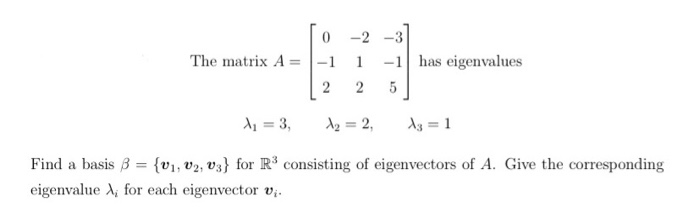0 -2 - The matrix A -11 2 2 -1 has eigenvalues 5 X = 3, A2 = 2, 13 = 1 Find a basis B = {V1, V2, v3} for R3 consisting of eigenvectors of A. Give the corresponding eigenvalue for each eigenvector vi.

• ### 4. (15 pts Consider the following direction fields IV VI (5 pts)Which of the direction fields corresponds to the system x -Ax, where A is a 2x2 matrix with eigenvalues λ,--1 and λ2-2 and correspondin...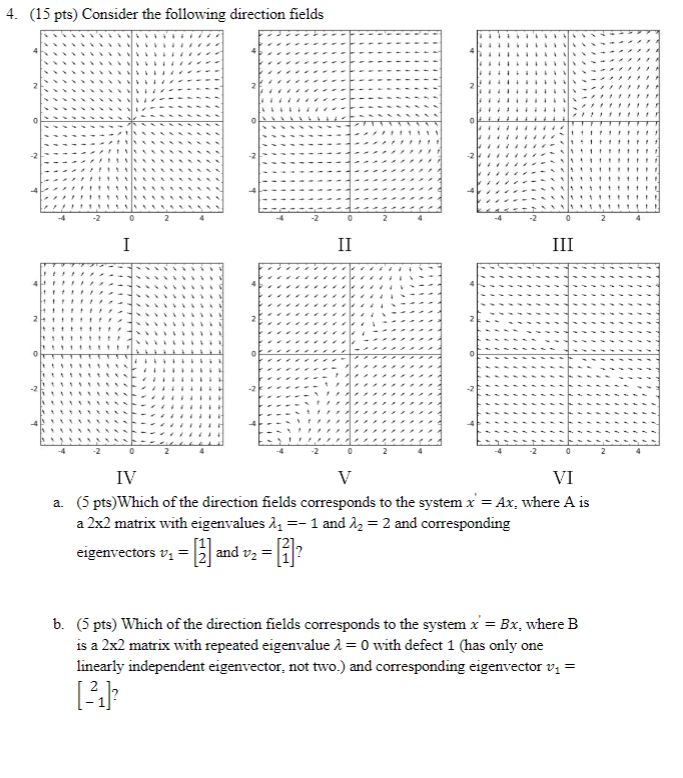4. (15 pts Consider the following direction fields IV VI (5 pts)Which of the direction fields corresponds to the system x -Ax, where A is a 2x2 matrix with eigenvalues λ,--1 and λ2-2 and corresponding eigenvectors vand v- 1? a. is a 2x2 matrix with repeated eigenvalue λ = 0 with defect 1 (has only one linearly independent eigenvector, not two.) and corresponding eigenvector vi- 13 (5 pts) Which of the direction fields corresponds to the system x -Cx, where...

• ### a) suppose that the nxn matrix A has its n eigenvalues arranged in decreasing order of absolute size, so that  >>....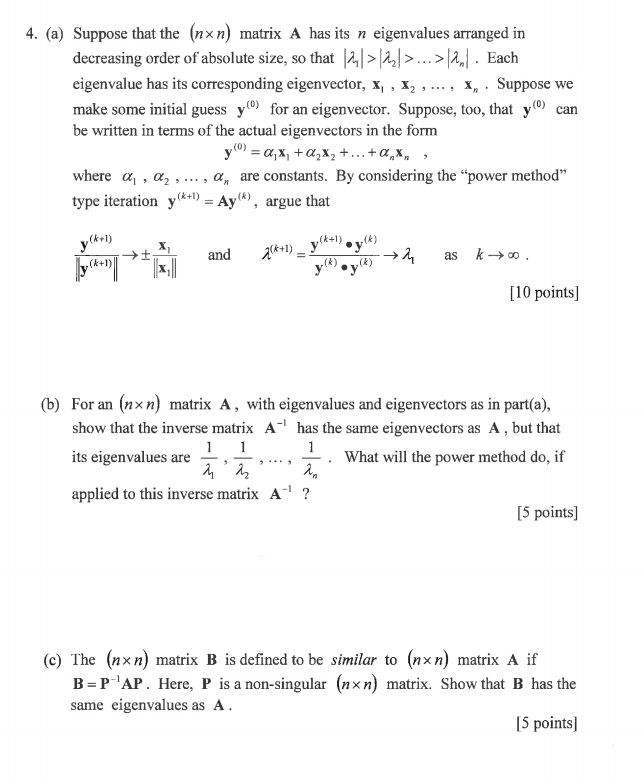a) suppose that the nxn matrix A has its n eigenvalues arranged in decreasing order of absolute size, so that  >>.... each eigenvalue has its corresponding eigenvector, x1,x2,...,xn. suppose we make some initial guess y(0) for an eigenvector. suppose, too, that y(0) can be written in terms of the actual eigenvectors in the form y(0)=alpha1.x1 +alpha2.x2 +...+alpha(n).x(n), where alpha1, alpha2, alpha(n) are constants. by considering the "power method" type iteration y(k+1)=Ay(k) argue that (see attached image) b) from an nxn...

• ### (1 point) Consider the linear system "(-1: 1) y. a. Find the eigenvalues and eigenvectors for...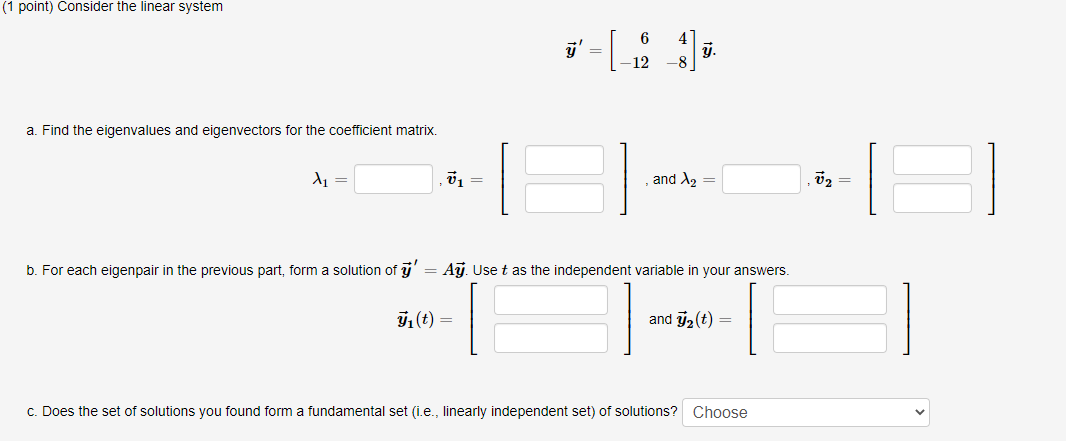(1 point) Consider the linear system "(-1: 1) y. a. Find the eigenvalues and eigenvectors for the coefficient matrix. 1 v1 = and 2 V2 b. For each eigenpair in the previous part, form a solution of y' = Ay. Use t as the independent variable in your answers. (t) = and yz(t) c. Does the set of solutions you found form a fundamental set (i.e., linearly independent set) of solutions? Choose

Free Homework App